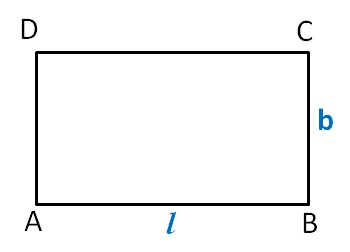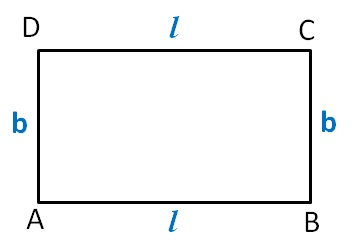Perimeter as variable

Chapter 11 Class 6 Algebra
Concept wise

A Rectangle has 4 sides

and opposite sides of a Rectangle are equal.Let AB = l & BC = b

So, CD = AB = l

& AD = BC = bThus,

Perimeter = Sum of all sides

= AB + BC + CD + AD

= l + b + l + b

= 2 l + 2b

= 2( l + b)

So we can write,

Perimeter of rectangle = 2( l + b)

where l is length and b is width of rectangle

Learn in your speed, with individual attention - Teachoo Maths 1-on-1 Class